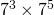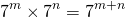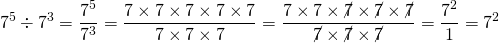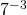# Exponent Laws

Classroom handout Exponent Laws

Handout Exponent Laws Solutions

Let’s examine. On this page, our values forare integers – a negative whole number, zero or a positive whole number.

We have defined the exponent to be a short hand for repeated multiplication.

It is easier to explain the laws with integer values, however an exponent can also be rational; irrational; or complex. We use irrational exponents whenever we define an exponential function over a real domain. On this page, we’ll look for the pattern made by integer exponents.

A summary of the laws can be found here.

# The Product Law

Now we know that. Let’s write that in another way:orWhat we notice about the indices is that they add up to four.

Let’s take a second example: suppose we have.

Writing that out in full give us:.

This leads us to the law for the product of two powers with the same base: for any values,,Of course, the base doesn’t have to be 7; 7 is used here just for illustration.

# The Quotient Law

Do you remember that a fraction is just a division? For example:We also know that when a fraction has a common factor on numerator and denominator, it can be simplified. For example:Usually, we don’t write out all of those steps. You might be more familiar with ‘scoring out’ or ‘cancelling’. Showing the steps without scoring out shows why scoring out works mathematically.

Let’s see how simplifying fractions helps with exponents. This time, let’s use ‘scoring out’.Or, in otherwords, we see a common factor ofon the numerator and the denominator. How many 7’s did we ‘score out’? We started with 5, we scored out 3, and we were left with 2. This leads us to the law for the quotient of two powers with the same base:or, more generally,# Negative Indices

The quotient law leads us to negative indices. Suppose we have.In summary,Or, in generalWhen we saywe mean,. When we saywe mean one divided bythree times over, that is:which is really awkward notation and is not often used. Better to say:# Exponent Zero

Now suppose we have. Well, we already know that anything divided by itself is just 1.

Let’s see what happens when we apply the quotient law:In general,(with the curious exception of a=0, but only sometimes…).

# The Power Law

The power law tells us what do when we have a power of a power:In short,.

# Distribution over a Product or Quotient

Now suppose we have the number, we know the answer is 100. Let’s write the number 10 as a product and then square it:In other words,This example illustrates that, in general:Similarly, with division:In general,Take Care though – an exponent does not distribute over addition/subtraction!

Example:The common mistake would be to say thatis equal tobut lets figure that out:which is not 49, so that is simply wrong.

# Summary

Click to open the summary!

Number Sense Question:

What might the value ofbe? What meaning does it have? If the math on this page extends to exponents that are not integers, what is the value of?

What about? Does it have any meaning?

See if your calculator can make sense ofand.